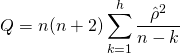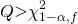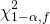## Ljuang-Box test:

The Ljung-Box test is used to check for autocorrelation in time series. The null hypothesis, H0, of this test is the absence of autocorrelation, so that the data are distributed independently. In our case we apply on the residues to check for the existence of this autocorrelation.

The alternative hypothesis in this case is the distribution of data dependent manner.

The statistic used in this case is:where n is the sample size delay, &rhocirc;2 is the autocorrelation of the sample in the k delay and h is the number of delays that are being tested.

For a significance level α, the critical region for rejection of the hypothesis of randomness is:whereis the α-quantile of the chi-square distribution with degrees of freedom h.

We use this possibility to reject the null hypothesis as a criterion to determine if the residuals are independent of each other and therefore if model obtained is valid. In our case it has taken a confidence interval of 95% so if we get p-values in this test lower than 0.05 reject the hypothesis and therefore residues are not independent of each other, so it is necessary to continue studying phenomena, unwanted, possibly you are on our data.

This test is commonly used to adjust ARIMA models, and to discard models that do not obtain independent residues feature present in the univariate stationary process desired, ruling out components such as trend or seasonality.

In this work we have applied the test of Ljung-Box on the "residuals" of the models created in order to rule out the presence of components of trend and seasonality in these models. Ruling out the presence of these components in the models, with a confidence interval of 95%, we can say that these models are not affected by such behavior components and correct them can get results.

## Teräsvirta test:

Reference test used to study the existence of a linear behavior in the data. Checking the linearity of the data is a particularly important preliminary step before using nonlinear models. The null hypothesis of this test is the linearity of the data studied. If the null hypothesis is not rejected you should use an autoregressive model to adjust the series.

The test is based on a single hidden-layer feedforward neural network. It performs a regression of a Taylor series expansion of the non-linear activation function of the neural network to the data. Then, it tests the null hypothesis of linearity of the data by testing if the coefficients of the non-linear terms of this expansion are zero. One of the main reasons for their selection, besides being a reference test in the field, is the power that has demonstrated against a lot of different non-linear behavior.

In our case it has taken a confidence interval of 95% so if we get p-values less than 0.05 in this test reject the null hypothesis and therefore it is advisable to use non-linear models to adjust the behavior of the data and to predict.

In this work we have applied the test datasets Teräsvirta on training in each case, can determine in this way, with a confidence interval of 95%, the presence of non-linear behavior in these datasets. Thus we can guess that the use of nonlinear models on these data is adequate.